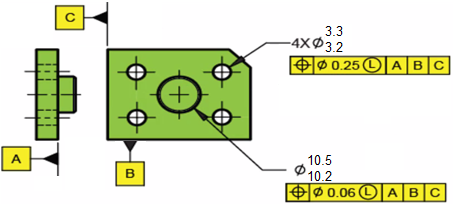## LMC Boundaries

Based on the two position tolerances shown, what are the respective outer and inner boundaries for the 4x holes and 1x pin?Hint
The Inner Boundary (IB) is a worst case theoretical boundary created by subtracting the stated geometric tolerance (and any other applicable tolerances) from the smallest allowed feature (LMC for for external features and MMC for internal ones).
Hint 2
The Outer Boundary (OB) is a worst case theoretical boundary created by adding the stated geometric tolerance (and any other applicable tolerances) from the largest allowed feature (MMC for external features and LMC for internal ones).
The Inner Boundary (IB) is a worst case theoretical boundary created by subtracting the stated geometric tolerance (and any other applicable tolerances) from the smallest allowed feature (LMC for for external features and MMC for internal ones). The Outer Boundary (OB) is a worst case theoretical boundary created by adding the stated geometric tolerance (and any other applicable tolerances) from the largest allowed feature (MMC for external features and LMC for internal ones).
Because there is a LMC feature modifier specified in the feature control frame, additional bonus tolerances need to be factored when determining IB and OB. In summary,
For an Internal Feature (Hole) with Geo Tol at LMC:
• IB = MMC (think smallest hole) - geo tol - bonus
• OB = LMC (think largest hole) + geo tol
For an External Feature (Pin) with Geo Tol at LMC:
• IB = LMC (think smallest pin) - geo tol
• OB = MMC (think largest pin) + geo tol + bonus
where $$geo\:tol$$ is the stated value in the feature control frame and $$bonus$$ is the max departure from the stated material condition.

Thus, the IB and OB for the hole are:
$$IB_{hole}=3.2-0.25-(3.3-3.2)=2.85$$$$$OB_{hole}=3.3+0.25=3.55$$$
Finally, the IB and OB for the pin are:
$$IB_{pin}=10.2-0.06=10.14$$$$$OB_{pin}=10.5+0.06+(10.5-10.2)=10.86$$$
$$IB_{hole}=2.85$$$$$OB_{hole}=3.55$$$
$$IB_{pin}=10.14$$$$$OB_{pin}=10.86$$$